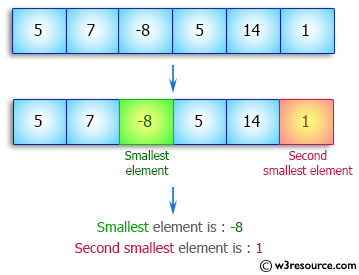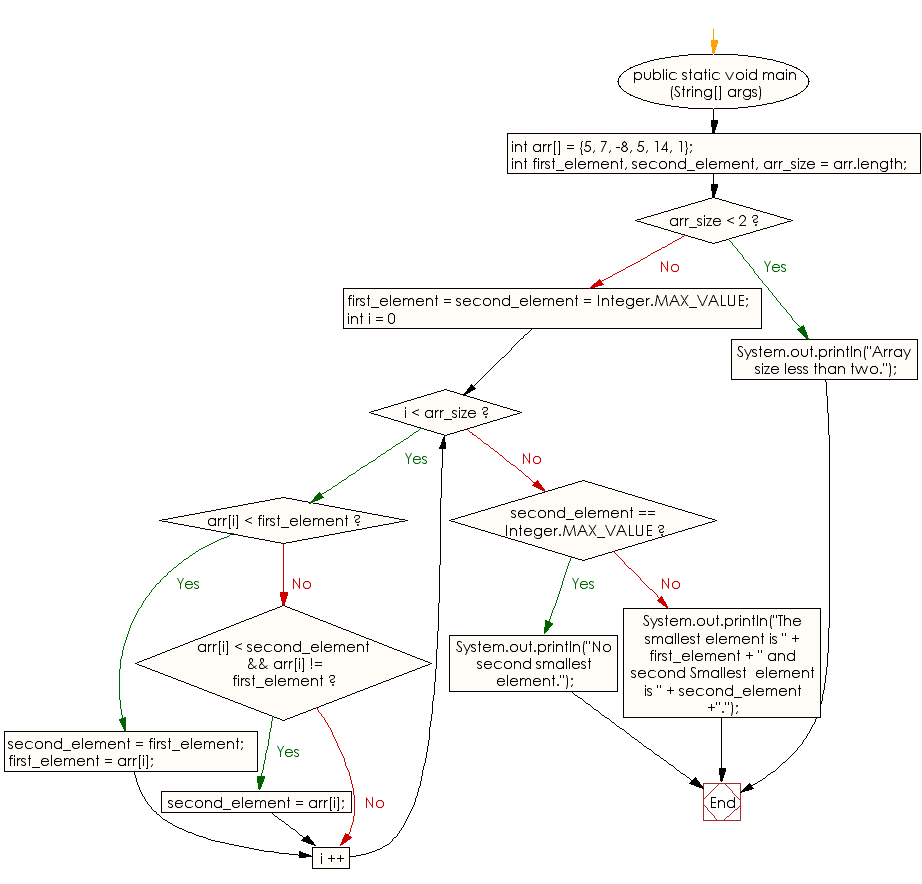﻿ Java: Find smallest and second smallest elements of an array# Java Array Exercises: Find smallest and second smallest elements of a given array

## Java Array: Exercise-41 with Solution

Write a Java program to find smallest and second smallest elements of a given array.

Pictorial Presentation:Sample Solution:

Java Code:

``````import java.util.*;
import java.lang.*;
public class Main
{
public static void main (String[] args)
{
int arr[] = {5, 7, -8, 5, 14, 1};

int first_element, second_element, arr_size = arr.length;

/* Return if the array size less than two */
if (arr_size < 2)
{
System.out.println("Array size less than two.");
return;
}

first_element = second_element = Integer.MAX_VALUE;
for (int i = 0; i < arr_size ; i ++)
{
/* Update both first and second if current element is smaller than first. */
if (arr[i] < first_element)
{
second_element = first_element;
first_element = arr[i];
}

/* Update second if arr[i] is between first and second
elements.*/
else if (arr[i] < second_element && arr[i] != first_element)
second_element = arr[i];
}
if (second_element == Integer.MAX_VALUE)
System.out.println("No second smallest element.");
else
System.out.println("The smallest element is " +
first_element + " and second Smallest  element is " + second_element +".");

}
}
```
```

Sample Output:

```
The smallest element is -8 and second Smallest  element is 1.
```

Flowchart:Visualize Java code execution (Python Tutor):

Java Code Editor:

Improve this sample solution and post your code through Disqus

What is the difficulty level of this exercise?

Test your Programming skills with w3resource's quiz.

﻿

## Java: Tips of the Day

countOccurrences

Counts the occurrences of a value in an array.

Use Arrays.stream().filter().count() to count total number of values that equals the specified value.

```public static long countOccurrences(int[] numbers, int value) {
return Arrays.stream(numbers)
.filter(number -> number == value)
.count();
}
```

Ref: https://bit.ly/3kCAgLb